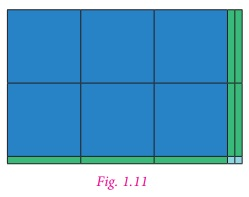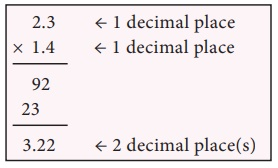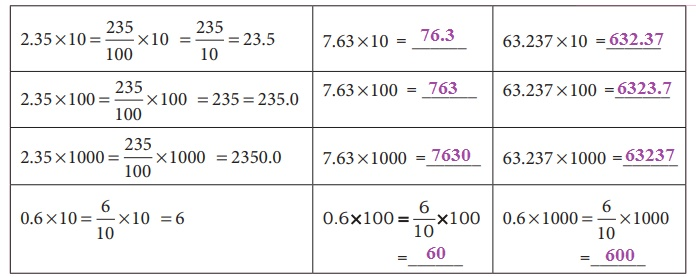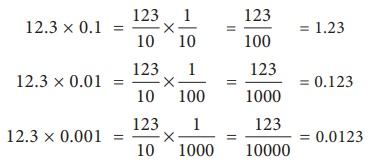Home | | Maths 7th Std | Multiplication of Decimal Numbers

# Multiplication of Decimal Numbers

(i) Decimal multiplication through models (ii) Multiplication of Decimal Numbers by 10, 100 and 1000

Operations on Decimal Numbers

Already we are familiar with decimal numbers. We know how to represent a decimal number as a fraction and the place values of digits. Now, let us learn the operations on decimal numbers.

Multiplication of Decimal Numbers

Mathan wants to buy a shirt material which costs ₹ 75.50 per metre. He needs 1.5 metre to stitch a shirt. How much does he have to pay? Here we need to multiply 75.50 and 1.5. In real life, there are many situations where we need to multiply decimal numbers.

(i) Decimal multiplication through models

Let us try to understand decimal multiplication using grid model.

Let us find 0.1 × 0.1.

0.1= 1/10 . Therefore, 0.1× 0.1= 1/10× 1/10

That is, 1/10 th of 1/10 .Shade horizontally 1/10 by blue colour (Fig. 1.7). Shade vertically 1/10 by green colour (Fig. 1.8).

Then 1/10 th of 1/10 is the common portion, that is 1/100 th .

Therefore, 1/10 × 1/10 = 1/100 = 0.01

Hence, 0.1 × 0.1 = 0.01.

Example 1.16

Find 0.3 × 0.4

Solution

First shade 4 rows of the grid in blue colour to represent 0.4. Shade 3 columns of the grid in green colour to represent 0.3 of 0.4. Now 12 squares represents the common portion. This represents 12 hundredth or 0.12. Hence 0.3 × 0.4 = 0.12.Note

The number of decimal digits in 0.12 is two. So, we can conclude that the number of decimal digits in the product of two decimal numbers is equal to the sum of decimal digits that are multiplied.

Area model

We have already learnt about the area model in the addition and subtraction of decimal numbers. In the same way we are going to multiply the decimal numbers. Now we shall see an example.

Example 1.17

Multiply 3.2 × 2.1

Solution

Let us try to represent the product of decimal numbers (3.2 × 2.1) as the area of a rectangle. Let us consider a rectangular portion as shown in Fig. 1.9.The rectangular portion is split into 3 wholes and 2 tenth along it’s length (Fig. 1.10).Since, 3 wholes and 2 tenth is multiplied with 2.1, we split the same area into 2 wholes and 1 tenth along its breadth (Fig. 1.11).Here, each row contains 3 wholes and 2 tenth. Each column contains 2 wholes and 1 tenth. The entire area model represents 6 wholes, 7 tenth and 2 hundredth.

Therfore, 3.2 × 2.1 = 6.72

Think

How are the products 2.1 × 3.2 and 21 × 32 alike? How are they different.

Solution:

2.1 × 3.2 = 6.72 and 21 × 32 = 672.

In both the cases the digits ambers are the same. But the place value differs.

Try these

(1) Shade the grid to multiply 0.3 × 0.6.Solution:3 rows of Yellow represent 0.3, 6 columns of Red colour represent 0.6 Double shaded 18 squares of orange colour represent.

0.30 × 0.6 = 0.18

(2) Use the area model to multiply 1.2 × 2.5Solution:Here each row contains 1 whole and 2 tenths. Each column contains 2 wholes and 5 tenths. The entire area model represents 2 wholes 9 tenths and 10 hundredths ( = 1 tenths). So 1.2 × 2.5 = 3.

Example 1.18

Multiply the following

(i) 2.3 × 1.4

(ii) 5.6 × 3.2

Solution

(i) 2.3 × 1.4

First, let us multiply 23 × 14

23×14=322

Now 2.3 × 1.4 = 3.22(ii) 5.6 × 3.2

First, let us multiply 56 × 32

56 × 32 =1792

Now 5.6 × 3.2 = 17.92Example 1.19

Latha purchased a churidhar material of 3.75 m at the rate of  ₹ 62.50 per metre. Find the amount to be paid.

Solution

Cost of churidhar material = ₹ 62.50 per metre

Length of churidhar material = 3.75 mAmount to be paid = 3.75 × 62.50

= ₹ 234.3750

= ₹ 234.38 (rounded to two decimals).

Example 1.20

The length and breadth of a rectangle is 23.5 cm and 1.5 cm respectively. Find the area of the rectangle.

Solution

Area of a rectangle = l × b sq. units

Here, l =23.5 cm, b=1.5 cm

Area of the rectangle = 23.5 × 1.5

= 35.25 sq.cm.(ii) Multiplication of Decimal Numbers by 10, 100 and 1000

We have studied about conversion of decimals into fractions in the first term. Consider 45.6 and 4.56.

Expressing these decimal numbers into fractions, we get

45.6 = 40 + 5 + 6/10 = 45 + 6/10 = 456/10

Now, 4.56 = 4 + 5/10 + 6/100= 456/100

Comparing the two fractions, we see that if there is one digit after the decimal point, then the denominator is 10 and if there are two digits after the decimal point, then the denominator is 100.

Let us see what happens if a decimal number is multiplied by 10, 100 and 1000.

Observe the following table and complete it.

Try theseCan you observe any pattern in the above table? There is a pattern in the shift of decimal points of the products in the table. In 2.35 × 10 = 23.5 the digits are the same, that is 2, 3, 5. Observe 2.35 and 23.5. To which side has the decimal point been shifted, right or left? The decimal point is shifted to the right by one place. Also note that 10 is one zero followed by 1.

In 2.35 × 100 = 235.0, observe 2.35 and 235. To which side and by how many digits has the decimal point been shifted? The decimal point has been shifted to the right by two places. Note that 100 is two zeros followed by 1.

Similarly in 2.35 × 1000 = 2350.0, we see that the decimal point has been shifted to the right by three places by adding one more digit 0 to the number 235. Note that 100 is three zeros followed by 1.

So we conclude that when a decimal number is multiplied by 10, 100 or 1000, the digits in the product are same as in the decimal number but the decimal point in the product is shifted to the right by as many places as there are zeros followed by 1.

Based on these observations we can now say,

0.02 × 10 = 0.2; 0.02 × 100 = 2 and 0.02 × 1000 = 20.

Can you find the following?

2.76×10=?

2.76 × 100 = ?

2.76 × 1000 = ?

Try these

Find

(i) 9.13×10 = 91.3

(ii) 9.13×100 = 913

(iii) 9.13×1000 = 9130

Example 1.21

Find the value of the following

(i) 3.26×10

(ii) 3.26×100

(iii) 3.26×1000

(iv) 7.01×10

(v) 7.01×100

(vi) 7.01×1000

Solution

(i) 3.26 × 10 = 32.6

(ii) 3.26 × 100 = 326.0

(iii) 3.26 × 1000 = 3260.0

(iv) 7.01 × 10 = 70.1

(v) 7.01 × 100 = 701.0

(vi) 7.01 × 1000 = 7010.0

Example 1.22

A concessional entrance ticket for students to visit a zoo is ₹ 12.50. How much has to be paid for 20 tickets?

Solution

Cost of one ticket = ₹ 12.50

Amount to be paid for 20 students = 12.50 × 20 = ₹ 250.00

We have already discussed about the multiplication of decimal numbers by 10, 100 and 1000. In the same way we can find patterns for multiplying decimal numbers by 0.1, 0.01 and 0.001. Observe the following.From the above multiplication we can conclude that, when multiplying by

•  0.1, the decimal point moves one place left.

•  0.01, the decimal point moves two places left.

•  0.001, the decimal point moves three places left.

Zeros may be needed after the decimal point as they are needed before the decimal point of numbers when multiplied 0.1, 0.01 and 0.0001.

Try this

Complete the following table :Tags : Number System | Term 3 Chapter 1 | 7th Maths , 7th Maths : Term 3 Unit 1 : Number System
Study Material, Lecturing Notes, Assignment, Reference, Wiki description explanation, brief detail
7th Maths : Term 3 Unit 1 : Number System : Multiplication of Decimal Numbers | Number System | Term 3 Chapter 1 | 7th Maths